# MA.912.GR.3.3Export Print
Use coordinate geometry to solve mathematical and real-world geometric problems involving lines, circles, triangles and quadrilaterals.

### Examples

Example: The line x+2y=10 is tangent to a circle whose center is located at (2,-1). Find the tangent point and a second tangent point of a line with the same slope as the given line.

Example: Given M(-4,7) and N(12,-1),find the coordinates of point P on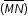so that P partitionsin the ratio 2:3.

### Clarifications

Clarification 1: Problems involving lines include the coordinates of a point on a line segment including the midpoint.

Clarification 2: Problems involving circles include determining points on a given circle and finding tangent lines.

Clarification 3: Problems involving triangles include median and centroid.

Clarification 4: Problems involving quadrilaterals include using parallel and perpendicular slope criteria.

General Information
Subject Area: Mathematics (B.E.S.T.)
Strand: Geometric Reasoning
Status: State Board Approved

## Benchmark Instructional Guide

• Circle
• Diameter
• Slope
• Triangle

### Vertical Alignment

Previous Benchmarks

Next Benchmarks

### Purpose and Instructional Strategies

In grade 8 and Algebra 1, students used coordinate systems to study lines and the find distances between points. In Geometry, students expand on their knowledge of coordinate geometry to solve problems geometric problems in real-world and mathematical contexts. In later courses, coordinates will be used to solve a variety of problems involving many shapes, including conic sections and shapes that can be studied using polar coordinates.
• Problem types include finding the midpoint of a segment (midpoint formula); partitioning a segment given endpoints and a ratio; writing the equation of a line, including lines that are parallel or perpendicular; finding the coordinates of the centroid of a triangle; and finding the distance between two points. In some cases, students may need to utilize systems of equations in order to determine solutions.
• Instruction includes the definition of a tangent to a circle and its properties, and the definition of the medians of a triangle and their point of concurrency (centroid).
• Instruction includes various approaches when finding the coordinates of a point partitioning a directed line segment (given the endpoints). (MTR.2.1MTR.3.1) Different methods are described below.
• The first concept that can be discussed is the connection to weighted average of two points. If the given ratio is a: b, that means the weights of the endpoints ($x$1 + $x$1) and ($x$2 + $y$2) are $\frac{\text{b}}{\text{a+b}}$ and $\frac{\text{a}}{\text{a+b}}$, respectively.
• For example, if the given ratio is 2: 3 and the points are at A(−3, 6) and B(4,−8) discuss with students where P is on its way from A to B. Students should be able to come with $\frac{\text{2}}{\text{5}}$. That means the weight of A is $\frac{\text{3}}{\text{5}}$ and the weight of B is $\frac{\text{2}}{\text{5}}$ . The next step is to calculate the $x$-coordinate of P using the weighted averages:  $x$p $\frac{\text{3}}{\text{5}}$x1 + $\frac{\text{2}}{\text{5}}$$x$2 which is equivalent to  $x$$\frac{\text{3}}{\text{5}}$(−3) + $\frac{\text{2}}{\text{5}}$(4) which is equivalent to $x$p = −$\frac{\text{1}}{\text{5}}$. Then calculate the $y$-coordinate of P using the weighted averages:  $y$p = $\frac{\text{3}}{\text{5}}$$y$1 + $\frac{\text{2}}{\text{5}}$$y$2 which is equivalent to $y$p = $\frac{\text{3}}{\text{5}}$(6) + $\frac{\text{2}}{\text{5}}$(−8).  Therefore, P is at (−$\frac{\text{1}}{\text{5}}$ , $\frac{\text{2}}{\text{5}}$).
• The second method uses the computations of a fraction of the horizontal and the vertical distance between the endpoints (partial distances). That is, if P is partitioning the segment in the ratio a : b or $\frac{\text{a}}{\text{a+b}}$ of the way from ($x$1 , $y$1) to ($x$2 , $y$2 then its location is $\frac{\text{a}}{\text{a+b}}$ of the horizontal distance and $\frac{\text{a}}{\text{a+b}}$ of the vertical distance from ($x$1 , $y$1) to ($x$2 , $y$2).
• For example, if the given ratio is 2: 3 and the points are at A(−3, 6) and B(4,−8) discuss with students where P is on its way from A to B. Students should be able to determine the horizontal distance from A to B as $x$2$x$ = 4 − (−3), which is 7. Then, determine the vertical distance 2 as $y$2$y$1 = −8 − 6, which is −14. Since the ratio is 2: 3, P is $\frac{\text{2}}{\text{5}}$ of the way from A to B. Students can calculate $\frac{\text{2}}{\text{5}}$ of the horizontal and the vertical distance, $\frac{\text{2}}{\text{5}}$(7) = $\frac{\text{14}}{\text{5}}$  and $\frac{\text{2}}{\text{5}}$−14) = −$\frac{\text{28}}{\text{5}}$, respectively. Students should realize that they will need to add the partial distances to A. Therefore, the coordinates of P are (−3 + $\frac{\text{14}}{\text{5}}$, 6 − $\frac{\text{28}}{\text{5}}$) = (−$\frac{\text{1}}{\text{5}}$ , $\frac{\text{2}}{\text{5}}$).
• Other methods include the use of formulas. It is important to note that this would require students to memorize formulas and not encourage students to explore all of the concepts.
• Instruction include that understanding that the midpoint of a segment partitions that segment in the ratio 1:1.
• Instruction includes various approaches when finding medians or centroids of triangles. (MTR.2.1, MTR.3.1) Different methods are described below.
• The equation of the line containing a median can be written from the coordinates of the vertex and the coordinates of the midpoint of the opposite side. Solving a system of equations formed by the equations of the two lines containing two medians of a triangle will result in the coordinates of the centroid.
• The centroid formula can be given as Centroid =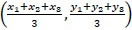, where ($x$1 , $y$1), ($x$2 , $y$2) and ($x$3 + y3) are the coordinates of the vertices of the triangle. Connections should be made to the fact that the coordinates of the centroid are the means of the coordinates of the vertices.
• The centroid is also the center of gravity of the triangle. Show them with a cardboard triangle that the triangle balances perfectly on its centroid (use a pencil or the tip of your finger).
• A method that makes connections to partitions can be found in the Centroid 2 Theorem. This theorem states that the centroid is of the distance from each 3 vertex to the midpoint of the opposite side, that is, the centroid partitions the 2 median of the way from the vertex to the midpoint of the opposite side. This 3 theorem can also be used to find the coordinates of centroid.
• For example, given a triangle with vertices A($x$1 + $y$1), B($x$2 + $y$2) and C($x$3 + $y$3), the midpoints of the sides will be A(a1 + b1) , N(a2 + b2) and L(a3 + b3) for  BC, AC and AB, respectively. Applying the Centroid Theorem to the median LC, the centroid is at ($\frac{\text{1}}{\text{3}}$$x$3 + $\frac{\text{2}}{\text{3}}$a3, $\frac{\text{1}}{\text{3}}$$y$3 + $\frac{\text{2}}{\text{3}}$b3). Using Substitution property of equality,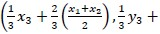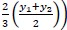=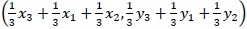. This results in Centroid =.

### Common Misconceptions or Errors

• Students may confuse using vertical and horizontal distances when partitioning segments.
• Students may need to be reminded that the segment partition refers to the parts, whose sum is the whole, not a part of a whole.
• For example, a segment partitioned in a 1:4 ratio is actually 5 parts separated into 1 part and 4 parts, not 1 out of 4 parts.

• What are the coordinates of the point that partitions segment AB in the ratio 2: 3?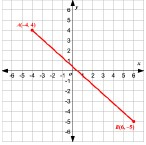• Circle A has center located at (2, 2) and contains the point (4,4).
• Part A. Write the equation that describes circle A
• Part B. Write the equation of a line tangent to Circle A at (4,4).
• Part C. Find the equation of a vertical tangent line and of a horizontal tangent line.

• Triangle ABC has two of its the vertices located at (−4,−1) and (3,−3).
• Part A. Triangle ABC has a centroid located at (−1, $\frac{\text{1}}{\text{3}}$). What is the third vertex of the triangle?
• Part B. Determine whether triangle ABC is a right triangle based on its angle measures and side lengths.
• Part C. If triangle ABC is not a right triangle, can you classify what type of triangle ABC is?

### Instructional Items

Instructional Item 1
• Given J(−4,2) and K(2,1), find the coordinates of point M on JK that partitions the segment into the ratio 1: 2.

*The strategies, tasks and items included in the B1G-M are examples and should not be considered comprehensive.

## Related Courses

This benchmark is part of these courses.
1200400: Foundational Skills in Mathematics 9-12 (Specifically in versions: 2014 - 2015, 2015 - 2022, 2022 and beyond (current))
1206310: Geometry (Specifically in versions: 2014 - 2015, 2015 - 2022, 2022 and beyond (current))
1206320: Geometry Honors (Specifically in versions: 2014 - 2015, 2015 - 2022, 2022 and beyond (current))
1206315: Geometry for Credit Recovery (Specifically in versions: 2014 - 2015, 2015 - 2022, 2022 and beyond (current))
7912065: Access Geometry (Specifically in versions: 2015 - 2022, 2022 and beyond (current))

## Related Access Points

Alternate version of this benchmark for students with significant cognitive disabilities.
MA.912.GR.3.AP.3: Use coordinate geometry to solve mathematical geometric problems involving lines, triangles and quadrilaterals.

## Related Resources

Vetted resources educators can use to teach the concepts and skills in this benchmark.

## Formative Assessments

Partitioning a Segment:

Students are asked to find the coordinates of a point which partitions a segment in a given ratio.

Type: Formative Assessment

Centroid Coordinates:

Students are asked to find the coordinates of the centroid when given the ratio of a directed segment.

Type: Formative Assessment

Proving Slope Criterion for Perpendicular Lines - 2:

Students are asked to prove that if the slopes of two lines are both opposite and reciprocal, then the lines are perpendicular.

Type: Formative Assessment

Proving Slope Criterion for Perpendicular Lines - 1:

Students are asked to prove that the slopes of two perpendicular lines are both opposite and reciprocal.

Type: Formative Assessment

Proving Slope Criterion for Parallel Lines - Two:

Students are asked to prove that two lines with equal slopes are parallel.

Type: Formative Assessment

Proving Slope Criterion for Parallel Lines - One:

Students are asked to prove that two parallel lines have equal slopes.

Type: Formative Assessment

Midpoints of Sides of a Quadrilateral:

Students are asked to prove that the quadrilateral formed by connecting the midpoints of the sides of a given quadrilateral is a parallelogram.

Type: Formative Assessment

Type of Triangle:

Students are given the coordinates of three vertices of a triangle and are asked to use algebra to determine whether the triangle is scalene, isosceles, or equilateral.

Type: Formative Assessment

Diagonals of a Rectangle:

Students are given the coordinates of three of the four vertices of a rectangle and are asked to determine the coordinates of the fourth vertex and show the diagonals of the rectangle are congruent.

Type: Formative Assessment

Students are given the coordinates of the vertices of a quadrilateral and are asked to determine whether the quadrilateral could also be a parallelogram, rhombus, rectangle, square, or trapezoid.

Type: Formative Assessment

## Lesson Plan

Keeping Triangles in Balance: Discovering Triangle Centroid is Concurrent Medians:

In this lesson, students identify, analyze, and understand the Triangle Centroid Theorem. Students discover that the centroid is the point of concurrency for the medians of a triangle and recognize its associated usage with the center of gravity or barycenter. This set of instructional materials provides the teacher with hands-on activities using technology as well as paper-and-pencil methods.

Type: Lesson Plan

## Perspectives Video: Professional/Enthusiast

Using Geometry and Computers to make Art with CNC Machining:

See and see far into the future of arts and manufacturing as a technician explains computer numerically controlled (CNC) machining bit by bit.

Type: Perspectives Video: Professional/Enthusiast

## MFAS Formative Assessments

Centroid Coordinates:

Students are asked to find the coordinates of the centroid when given the ratio of a directed segment.

Students are given the coordinates of the vertices of a quadrilateral and are asked to determine whether the quadrilateral could also be a parallelogram, rhombus, rectangle, square, or trapezoid.

Diagonals of a Rectangle:

Students are given the coordinates of three of the four vertices of a rectangle and are asked to determine the coordinates of the fourth vertex and show the diagonals of the rectangle are congruent.

Midpoints of Sides of a Quadrilateral:

Students are asked to prove that the quadrilateral formed by connecting the midpoints of the sides of a given quadrilateral is a parallelogram.

Partitioning a Segment:

Students are asked to find the coordinates of a point which partitions a segment in a given ratio.

Proving Slope Criterion for Parallel Lines - One:

Students are asked to prove that two parallel lines have equal slopes.

Proving Slope Criterion for Parallel Lines - Two:

Students are asked to prove that two lines with equal slopes are parallel.

Proving Slope Criterion for Perpendicular Lines - 1:

Students are asked to prove that the slopes of two perpendicular lines are both opposite and reciprocal.

Proving Slope Criterion for Perpendicular Lines - 2:

Students are asked to prove that if the slopes of two lines are both opposite and reciprocal, then the lines are perpendicular.

Type of Triangle:

Students are given the coordinates of three vertices of a triangle and are asked to use algebra to determine whether the triangle is scalene, isosceles, or equilateral.

## Student Resources

Vetted resources students can use to learn the concepts and skills in this benchmark.

## Perspectives Video: Professional/Enthusiast

Using Geometry and Computers to make Art with CNC Machining:

See and see far into the future of arts and manufacturing as a technician explains computer numerically controlled (CNC) machining bit by bit.

Type: Perspectives Video: Professional/Enthusiast

## Parent Resources

Vetted resources caregivers can use to help students learn the concepts and skills in this benchmark.

## Perspectives Video: Professional/Enthusiast

Using Geometry and Computers to make Art with CNC Machining:

See and see far into the future of arts and manufacturing as a technician explains computer numerically controlled (CNC) machining bit by bit.

Type: Perspectives Video: Professional/Enthusiast